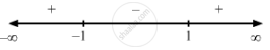Share

F(X) = X3 − 3x . - CBSE (Science) Class 12 - Mathematics

Question

f(x) = x$-$ 3x .

Solution

$\text { Given: } \hspace{0.167em} f\left( x \right) = \left( x - 5 \right)^4$

$\Rightarrow f'\left( x \right) = 4 \left( x - 5 \right)^3$

$\text { For a local maximum or a local minimum, we must have }$

$f'\left( x \right) = 0$

$\Rightarrow 4 \left( x - 5 \right)^3 = 0$

$\Rightarrow x = 5$Since f '(x) changes from negative to positive as x increases through 1, x = 1 is the point of local minima.
The local minimum value of  f (x) at x = 1 is given by $\left( 1 \right)^3 - 3\left( 1 \right) = - 2$

Since f '(x) changes from positive to negative when x increases through -1, x = -1 is the point of local maxima.
The local maximum value of  f (x) at x = -1 is given by $\left( - 1 \right)^3 - 3\left( - 1 \right) = 2$

Is there an error in this question or solution?

Video TutorialsVIEW ALL 

Solution F(X) = X3 − 3x . Concept: Graph of Maxima and Minima.
S